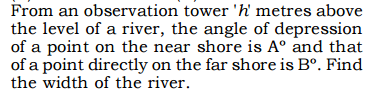## Maths Quiz for SSC CGL Tier-2 with Complete Explanation

Maths is a scoring paper in SSC CGL Tier-2 Exam. Many students score more than 190 out of 200 that help them to grab their dream job. As you all know, SSC CGL Tier-2 2016 is most likely to be conducted in second week of November, you hardly have 1 month for mastering this subject. To help you, we are providing you a Maths Quiz for SSC CGL Tier-2 2016. This quiz comprises 10 questions. Try to solve these questions as fast as you can.

We will keep posting more Maths quizzes for SSC CGL Tier-2. So, keep visiting us.

We have already provided some Maths Quizzes for SSC CGL Exam. If you want to check those quizzes, click on below given links:

# Maths Quiz for SSC CGL Tier-2

• #### 1.1. (b-1)/(b+2)
2. (b-1)/(b+1)
3. (b2-1)/(b2+1)
4. (b2-2)/(b2+1)
Explanation:
Note:

# Maths Quiz for SSC CGL Tier-2

• #### 2.1. h (Cot B + Cot A)
2. h (Tan B + Tan A)
3. h (Tan A - Tan B)
4. h (Cot B- Cot A)
Explanation:
Note:

1. Rs 2600
2. Rs 2680
3. Rs 2580
4. Rs 2500
Explanation:
Note:

1. 2
2. 3
3. 1
4. 0
Explanation:
Note:

# Maths Quiz for SSC CGL Tier-2

• #### 5.1. 2/ (1+a)
2. 2/(1-a)
3. 1/(1+a)
4. None of these
Explanation:
Note:

# Maths Quiz for SSC CGL Tier-2

• #### 6.1. abc
2. 3abc
3. 0
4. None of these
Explanation:
Note:

1. 30.77%
2. 74.00%
3. 25.16%
4. 88.40%
Explanation:
Note:

# Maths Quiz for SSC CGL Tier-2

• #### 8. A man rides at the rate of 300 Metres per minute and stops for 6 minutes to change horses at the end of every sixth Kilometre. Then to travel a distance of 84 km, the time taken by the man is

1. 3 hours 15 minutes
2. 6 hours 17 minutes
3. 5 hours 18 minutes
4. 2 hours 17 minutes
Explanation:
Note: Speed of horse rider = 350 metre/minute = 21 km/h

84/6 = 14 . It means, he will change the horse 13 times.

Time taken in changing the horses = 13 x 6 = 78 minutes
Time taken in covering 84 km = 4 hours
Total time = 4 hours + 78 minutes
= 5 hours 18 minutes

1. x-z
2. 2x-z
3. x+z
4. 2z-x

1. 12.5%
2. 18%
3. 20%
4. 16.66%
Explanation:
Note: